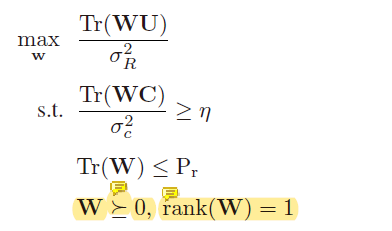hello,I now want to use this optimization problem to get the best W, but the result has always been infeasible. Excuse me, what’s wrong with this code？
this is the optimization problemand this is my codes

clc,clear,close all
M = 20;
Mx = 4;
Mz = 5;
Nx = 3;
Nz = 4;
N = Nx*Nz;
sigama_r = 0.1;
sigama_c = 0.1;
Pr = 3;
SNR_cu = 10^(22/10);

pos_bs = [0,2];
pos_ris = [100,2];
pos_cu = [100,1];

d_br = norm(pos_bs-pos_ris,2);
d_ru = norm(pos_ris-pos_cu,2);
d_bu = norm(pos_cu-pos_bs,2);
L = 3;

G = generate_channel_BR(Mx,Mz,Nx,Nz,L,d_br);
hd = generate_channel_BU(Mx,Mz,L,d_bu);
hr = generate_channel_RU(Nx,Nz,L,d_ru);
[A,B] = generate_AB(M,N);
V = diag(exp(1j*(rand(1,N)2pi)));

U1 = G’VA*V’*G+B;
U = U1’*U1;
C1 = hd’+hr’*V’*G;
C = C1’*C1;

cvx_begin sdp
variable W(M, M)
maximize(real(trace(WU))/sigama_r);
subject to
real(trace(W
C))/sigama_c >= SNR_cu;
real(trace(W)) <= Pr;
W == hermitian_semidefinite(M);
cvx_end

This is the result of the operation：

## Calling SDPT3 4.0: 402 variables, 192 equality constraints

num. of constraints = 192
dim. of sdp var = 40, num. of sdp blk = 1
dim. of linear var = 2

SDPT3: Infeasible path-following algorithms

## number of iterations = 6 residual of primal infeasibility certificate (y,Z) = 2.00e-22 reldist to infeas. <= 4.11e-21 Total CPU time (secs) = 0.24 CPU time per iteration = 0.04 termination code = 1 DIMACS: 4.7e+02 0.0e+00 1.8e-02 0.0e+00 -1.0e+00 5.0e-04

Status: Infeasible
Optimal value (cvx_optval): -Inf

This is technically possible because I’m currently redoing the paper, but I don’t know what has been causing the problem. Could you take a look at it for me, Please

The post below has suggestion for what to do:

Hi,Erling,
I want to know something about input data scaling. When some data have e-7 order of magnitudes while other data have e+07 order of magnitudes, do I still need to do the data scaling? Because when data is scaled too much, some features have been droped.

Input data varying from e-7 to e7 spans 14 orders of magnitude (digits), out of the approximately 16 digits available to a double precision solver. That is likely to result in numerical difficulties or unreliability.

We don’t know much about your problem. If there is not a way to scale your inputs such that they span a much smaller range, perhaps some of the features should be dropped? Or if all those features are really important to the problem, maybe you need a higher precision (for instance, quad precision) solver, of which there are some for certain types of problems, but not available under CVX.